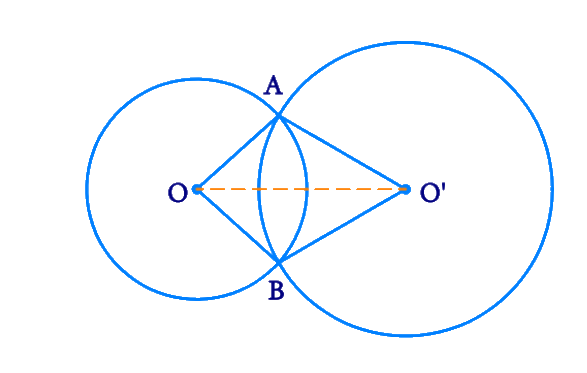# Ex.10.6 Q1 Circles Solution - NCERT Maths Class 9

## Question

Prove that the line of centres of two intersecting circles subtends equal angles at the two points of intersection.

Video Solution
Circles
Ex 10.6 | Question 1

## Text Solution

What is known?

Two intersecting circles.

What is unknown?

To prove that the line of centres of two intersecting circles subtends equal angles at the two points of intersection

Reasoning:

Using Side-Side-Side (SSS criteria) and Corresponding parts of congruent triangles (CPCT) we prove the statement.

Steps:

Draw $$2$$ intersecting circles with centres $${O}$$ and $${O’}$$ respectively. Join these $$2$$ centres.

Let the points of intersection be $${A}$$ and $${B.}$$We need to prove that

\begin {align}\angle {OAO}^{\prime}=\angle {OBO}^{\prime} \end {align}

Consider \begin {align}\Delta {OAO}^{\prime} \end {align} and \begin {align}\Delta {OBO}^{\prime} \end {align}

\begin{align}{OA}&={OB}\\ \text { (Radii of circle}&\text{ with centre O)}\\\\ {O}^{\prime} {A}&={O}^{\prime } {B} \\ \text { (Radii of circle} & \text {with centre O^\prime )} \\\\{OO}^{\prime}&={O} {O}^{\prime} \\&\text { (Common) }\end{align}

Therefore by SSS criteria, \begin {align}\Delta {O} {AO}^{\prime} \end {align} and \begin {align}\Delta {O} {BO}^{\prime} \end {align} are congruent to each other.

\begin {align}{\rm{By\,\, CPCT}} , \;\angle {OAO}^{\prime}=\angle {OBO}^{\prime} \end {align}

Hence it is proved that the line of centres of two intersecting circles subtends equal angles at the two points of intersection.

Video Solution
Circles
Ex 10.6 | Question 1
Learn from the best math teachers and top your exams

• Live one on one classroom and doubt clearing
• Practice worksheets in and after class for conceptual clarity
• Personalized curriculum to keep up with school